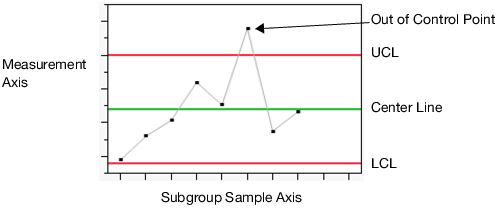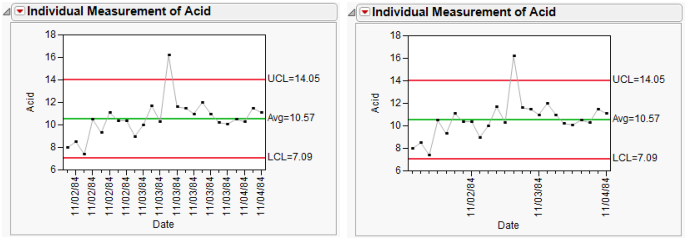Quality and Process Methods > Legacy Control Charts > Legacy Control Chart Reports
Publication date: 07/30/2020

# Legacy Control Chart Reports

The analysis produces a chart that can be used to determine whether a process is in a state of statistical control. The report varies depending on the type of chart that you select. Figure 12.7 displays the parts of a simple control chart. Control charts update dynamically as data is added or changed in the data table.

Figure 12.7 Example of a Control ChartNote: Any rows that are excluded in the data table are also hidden in Runs charts, P charts, U charts, and C charts.

Control charts have the following characteristics:

Each point plotted on the chart represents an individual process measurement or summary statistic. In Figure 12.7, the points represent the average for a sample of measurements.

Subgroups should be chosen rationally, that is, they should be chosen to maximize the probability of seeing a true process change between subgroups.

The vertical axis of a control chart is scaled in the same units as the summary statistic.

The horizontal axis of a control chart identifies the subgroup samples and is time ordered. Observing the process over time is important in assessing if the process is changing.

The green line is the center line, or the average of the data. The center line indicates the average (expected) value of the summary statistic when the process is in statistical control. Measurements should appear equally on both sides of the center line. If not, this is possible evidence that the process average is changing.

The two red lines are the upper and lower control limits, labeled UCL and LCL. These limits give the range of variation to be expected in the summary statistic when the process is in statistical control. If the process is exhibiting only routine variation, then all the points should fall randomly in that range. In Figure 12.7, one measurement is above the upper control limit. This is evidence that the measurement could have been influenced by a special cause, or is possibly a defect.

A point outside the control limits (or the V-mask of a CUSUM chart) signals the presence of a special cause of variation.

Options within each platform create control charts that can be updated dynamically as samples are received and recorded or added to the data table.

When a control chart signals abnormal variation, action should be taken to return the process to a state of statistical control if the process degraded. If the abnormal variation indicates an improvement in the process, the causes of the variation should be studied and implemented.

When you double-click the horizontal or vertical axis, the appropriate Axis Specification window appears for you to specify the format, axis values, number of ticks, gridline, reference lines, and other options to display on the axis.

For example, the Pickles.jmp data lists measurements taken each day for three days. In Figure 12.8, by default, the horizontal axis is labeled at every other tick. Sometimes this gives redundant labels, as shown to the left in Figure 12.8. If you specify a label at an increment of eight, the horizontal axis is labeled once for each day, as shown in the chart on the right.

Figure 12.8 Example of Labeled x Axis Tick MarksTip: For information about warnings and rules, see Tests and Westgard Rules in the Control Chart Builder section of this guide.